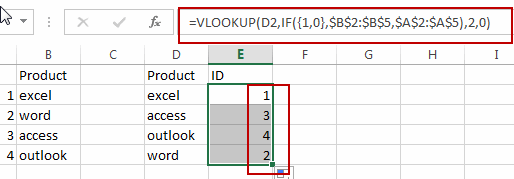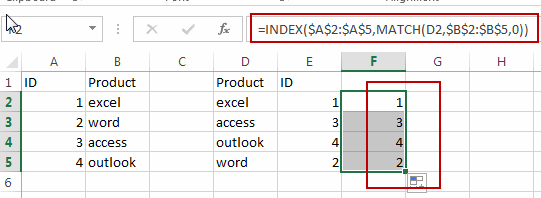# How to Lookup Values from Right to Left in Excel

This post will guide you how to vlookup values from right to left in your worksheet in excel. How do I look up values to left column with a column in Excel 2013/2016. How to use Vlookup function to extract the corresponding value of the left column in your worksheet in Excel.

## Lookup Values to Left Column

By default, you can use the VLOOKUP function to lookup values in a range and then return the corresponding value of the right column in Excel. And if you want to extract the corresponding value of the left column. How to do it.

Assuming that you have a list of data in range A1:B5, and you have product names in range D2:D5, and you want to get their relative ID values in the left Product column. You can use the following formula with VLOOKUP function to achieve it.

`=VLOOKUP(D2,IF({1,0},\$B\$2:\$B\$5,\$A\$2:\$A\$5),2,0)`

Type this formula into formula E2, and press Enter key, then drag the AutoFill Handle down to other cells to apply this formula. Let’s see the last result:Note: D2 is the value that you want to look up from range A1:B5. B2:B5 is a range that you want to looking for, A2:A5 is the range that contain the value that you want to extract.

You can also use another formula based on the INDEX function and the MATCH function to achieve the same result of extract values from right to left in Excel. Like this:

`=INDEX(\$A\$2:\$A\$5,MATCH(D2,\$B\$2:\$B\$5,0))`### Related Functions

• Excel INDEX function
The Excel INDEX function returns a value from a table based on the index (row number and column number)The INDEX function is a build-in function in Microsoft Excel and it is categorized as a Lookup and Reference Function.The syntax of the INDEX function is as below:= INDEX (array, row_num,[column_num])…
• Excel MATCH  function
The Excel MATCH function search a value in an array and returns the position of that item.The MATCH function is a build-in function in Microsoft Excel and it is categorized as a Lookup and Reference Function.The syntax of the MATCH function is as below:= MATCH  (lookup_value, lookup_array, [match_type])….
• Excel IF function
The Excel IF function perform a logical test to return one value if the condition is TRUE and return another value if the condition is FALSE. The IF function is a build-in function in Microsoft Excel and it is categorized as a Logical Function.The syntax of the IF function is as below:= IF (condition, [true_value], [false_value])….
• Excel VLOOKUP function
The Excel VLOOKUP function lookup a value in the first column of the table and return the value in the same row based on index_num position.The syntax of the VLOOKUP function is as below:= VLOOKUP (lookup_value, table_array, column_index_num,[range_lookup])….